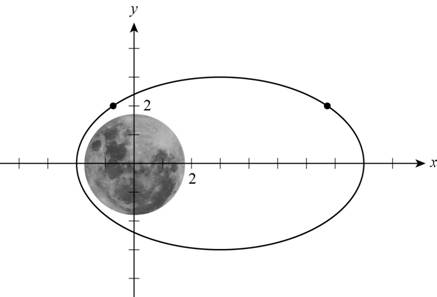# The closest and the farthest points that the satellite gets to the center of the moon in the graph.### Precalculus: Mathematics for Calcu...

6th Edition
Stewart + 5 others
Publisher: Cengage Learning
ISBN: 9780840068071### Precalculus: Mathematics for Calcu...

6th Edition
Stewart + 5 others
Publisher: Cengage Learning
ISBN: 9780840068071

#### Solutions

Chapter 1.8, Problem 115E

(a)

To determine

## The closest and the farthest points that the satellite gets to the center of the moon in the graph.

Expert Solution

The closest and the farthest points that the satellite gets to the center of the moon in the graph is (2,0) and (8,0) respectively.

### Explanation of Solution

Given:

The equation of satellite’s orbit is,

(x3)225+y216=1

And the graph of orbit with the centre of the moon at the origin is,Figure (1)

Calculation:

The closest and farthest points in satellite’s orbit can be found by the intersection points of equation (x3)225+y216=1 and y=0 (x-axis),

Substitute 0 for y in orbit equation (x3)225+y216=1 for the x,

(x3)225+0216=1(x3)225=1(x3)2=25x3=25

Further solve,

x3=25x=(±5+3)x=8,2

So the closest and the farthest points that the satellite gets to the center of the moon in the graph is (2,0) and (8,0) respectively.

(b)

To determine

### The x-coordinates of points in the orbit with y-coordinates 2 and find their distances to the center of moon.

Expert Solution

The x-coordinates of points in the orbit with y-coordinates 2 is 7.3303 and (1.3303) . and distance of the point (7.3303,2) with the center of moon (0,0) is 7.60megameters and distance of the point (1.3303,2) with the center of moon (0,0) is 2.40megameters.

### Explanation of Solution

Given:

The equation of satellite’s orbit is,

(x3)225+y216=1

And the graph of orbit with the centre of the moon at the origin is,Figure (1)

Calculation:

The x-coordinates of the points in the orbit with y-coordinates 2 is,

(x3)225+2216=1(x3)225+416=1(x3)225=114(x3)225=34

Further solve,

(x3)225=34(x3)2=34×25x3=754x=(±532+3)

So the two value of x is (532+3)=7.3303 and (532+3)=(1.3303) .

And the distance of the point (7.3303,2) with the center of moon (0,0) is,

(7.33030)2+(20)2=53.73+4=57.73=7.60

So distance of the point (7.3303,2) with the center of moon (0,0) is 7.60megameters.

And the distance of the point (1.3303,2) with the center of moon (0,0) is,

(1.33030)2+(20)2=1.77+4=5.77=2.40

So distance of the point (1.3303,2) with the center of moon (0,0) is 2.40megameters.

### Have a homework question?

Subscribe to bartleby learn! Ask subject matter experts 30 homework questions each month. Plus, you’ll have access to millions of step-by-step textbook answers!